## UGBA 198 - 3 Lecture 7¶

https://ugba198.org

## Decision Trees and Random Forests¶

In :
#importing the necessary packages
%matplotlib inline
import matplotlib.pyplot as plt
from mpl_toolkits import mplot3d
import seaborn as sns; sns.set() # seaborn is a library for creating nice visualizations

import numpy as np
import pandas as pd
import sklearn

In :
# using sklearn implementation of Decision Trees
from sklearn.tree import DecisionTreeClassifier
from sklearn.ensemble import RandomForestClassifier
from sklearn.model_selection import cross_val_score
from sklearn.metrics import confusion_matrix

/Users/Me/anaconda3/lib/python3.7/site-packages/sklearn/ensemble/weight_boosting.py:29: DeprecationWarning: numpy.core.umath_tests is an internal NumPy module and should not be imported. It will be removed in a future NumPy release.
from numpy.core.umath_tests import inner1d


### Example 2D data¶

In :
from sklearn.datasets import make_blobs

X, y = make_blobs(n_samples=300, centers=4,
random_state=0, cluster_std=1.0)
plt.scatter(X[:, 0], X[:, 1], c=y, s=50, cmap='rainbow');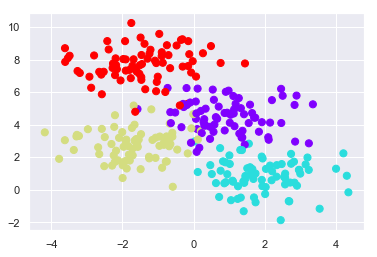In :
# Utility functions for visualizations ------
def visualize_tree(estimator, X, y, boundaries=True,
xlim=None, ylim=None, ax=None):
ax = ax or plt.gca()

# Plot the training points
ax.scatter(X[:, 0], X[:, 1], c=y, s=30, cmap='viridis',
clim=(y.min(), y.max()), zorder=3)
ax.axis('tight')
ax.axis('off')
if xlim is None:
xlim = ax.get_xlim()
if ylim is None:
ylim = ax.get_ylim()

# fit the estimator
estimator.fit(X, y)
xx, yy = np.meshgrid(np.linspace(*xlim, num=200),
np.linspace(*ylim, num=200))
Z = estimator.predict(np.c_[xx.ravel(), yy.ravel()])

# Put the result into a color plot
n_classes = len(np.unique(y))
Z = Z.reshape(xx.shape)
contours = ax.contourf(xx, yy, Z, alpha=0.3,
levels=np.arange(n_classes + 1) - 0.5,
cmap='viridis', clim=(y.min(), y.max()),
zorder=1)

ax.set(xlim=xlim, ylim=ylim)

# Plot the decision boundaries
def plot_boundaries(i, xlim, ylim):
if i >= 0:
tree = estimator.tree_

if tree.feature[i] == 0:
ax.plot([tree.threshold[i], tree.threshold[i]], ylim, '-k', zorder=2)
plot_boundaries(tree.children_left[i],
[xlim, tree.threshold[i]], ylim)
plot_boundaries(tree.children_right[i],
[tree.threshold[i], xlim], ylim)

elif tree.feature[i] == 1:
ax.plot(xlim, [tree.threshold[i], tree.threshold[i]], '-k', zorder=2)
plot_boundaries(tree.children_left[i], xlim,
[ylim, tree.threshold[i]])
plot_boundaries(tree.children_right[i], xlim,
[tree.threshold[i], ylim])

if boundaries:
plot_boundaries(0, xlim, ylim)

def plot_tree_interactive(X, y):
def interactive_tree(depth=5):
clf = DecisionTreeClassifier(max_depth=depth, random_state=0)
visualize_tree(clf, X, y)

return interact(interactive_tree, depth=[1, 5])

def randomized_tree_interactive(X, y):
N = int(0.75 * X.shape)

xlim = (X[:, 0].min(), X[:, 0].max())
ylim = (X[:, 1].min(), X[:, 1].max())

def fit_randomized_tree(random_state=0):
clf = DecisionTreeClassifier(max_depth=15)
i = np.arange(len(y))
rng = np.random.RandomState(random_state)
rng.shuffle(i)
visualize_tree(clf, X[i[:N]], y[i[:N]], boundaries=False,
xlim=xlim, ylim=ylim)

interact(fit_randomized_tree, random_state=[0, 100]);

def visualize_classifier(model, X, y, ax=None, cmap='rainbow'):
ax = ax or plt.gca()

# Plot the training points
ax.scatter(X[:, 0], X[:, 1], c=y, s=30, cmap=cmap,
clim=(y.min(), y.max()), zorder=3)
ax.axis('tight')
ax.axis('off')
xlim = ax.get_xlim()
ylim = ax.get_ylim()

# fit the estimator
model.fit(X, y)
xx, yy = np.meshgrid(np.linspace(*xlim, num=200),
np.linspace(*ylim, num=200))
Z = model.predict(np.c_[xx.ravel(), yy.ravel()]).reshape(xx.shape)

# Create a color plot with the results
n_classes = len(np.unique(y))
contours = ax.contourf(xx, yy, Z, alpha=0.3,
levels=np.arange(n_classes + 1) - 0.5,
cmap=cmap, clim=(y.min(), y.max()),
zorder=1)

ax.set(xlim=xlim, ylim=ylim)


### Decision trees of various depths¶

In :
fig, ax = plt.subplots(1, 4, figsize=(16, 3))

X, y = make_blobs(n_samples=300, centers=4,
random_state=0, cluster_std=1.0)

for axi, depth in zip(ax, range(1, 5)):
model = DecisionTreeClassifier(max_depth=depth)
visualize_tree(model, X, y, ax=axi)
axi.set_title('depth = {0}'.format(depth))

/Users/Me/anaconda3/lib/python3.7/site-packages/matplotlib/contour.py:1000: UserWarning: The following kwargs were not used by contour: 'clim'
s)
/Users/Me/anaconda3/lib/python3.7/site-packages/matplotlib/contour.py:1000: UserWarning: The following kwargs were not used by contour: 'clim'
s)
/Users/Me/anaconda3/lib/python3.7/site-packages/matplotlib/contour.py:1000: UserWarning: The following kwargs were not used by contour: 'clim'
s)
/Users/Me/anaconda3/lib/python3.7/site-packages/matplotlib/contour.py:1000: UserWarning: The following kwargs were not used by contour: 'clim'
s)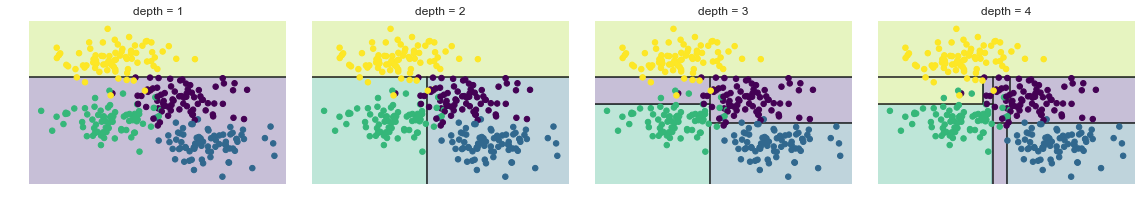In :
tree = DecisionTreeClassifier().fit(X, y)

In :
visualize_tree(tree, X, y)
print(tree.tree_.max_depth)

10

/Users/Me/anaconda3/lib/python3.7/site-packages/matplotlib/contour.py:1000: UserWarning: The following kwargs were not used by contour: 'clim'
s)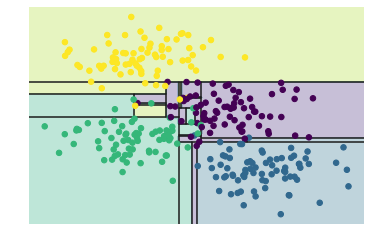### Overfitting decision tree¶

In :
# two models trained on different subsets of data
model = DecisionTreeClassifier()

fig, ax = plt.subplots(1, 2, figsize=(16, 6))
visualize_tree(model, X[::2], y[::2], boundaries=False, ax=ax)
visualize_tree(model, X[1::2], y[1::2], boundaries=False, ax=ax)

/Users/Me/anaconda3/lib/python3.7/site-packages/matplotlib/contour.py:1000: UserWarning: The following kwargs were not used by contour: 'clim'
s)
/Users/Me/anaconda3/lib/python3.7/site-packages/matplotlib/contour.py:1000: UserWarning: The following kwargs were not used by contour: 'clim'
s)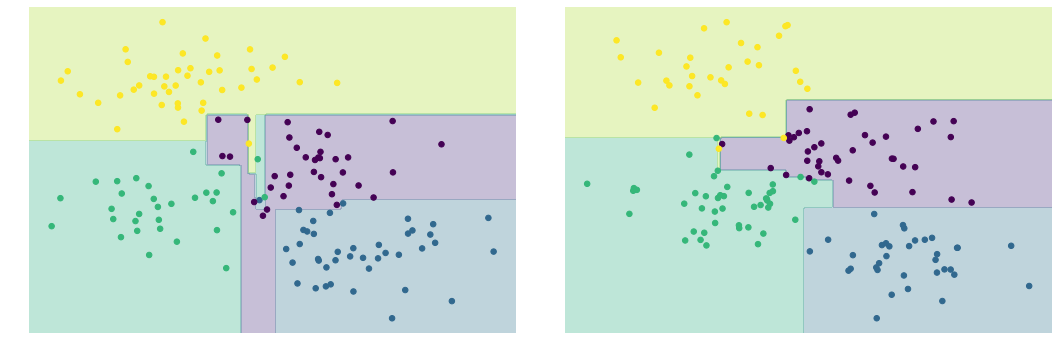### Random Forest Classifier¶

In :
tree = DecisionTreeClassifier()
forest = RandomForestClassifier(n_estimators=1)

forest.fit(X, y)
visualize_classifier(forest, X, y)

/Users/Me/anaconda3/lib/python3.7/site-packages/matplotlib/contour.py:1000: UserWarning: The following kwargs were not used by contour: 'clim'
s)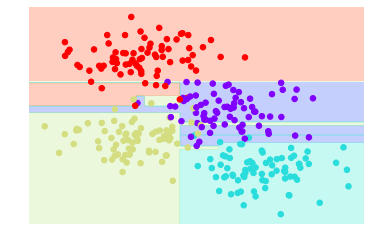In :
tree = DecisionTreeClassifier()
forest = RandomForestClassifier(n_estimators=10)

forest.fit(X, y)
visualize_classifier(forest, X, y)

/Users/Me/anaconda3/lib/python3.7/site-packages/matplotlib/contour.py:1000: UserWarning: The following kwargs were not used by contour: 'clim'
s)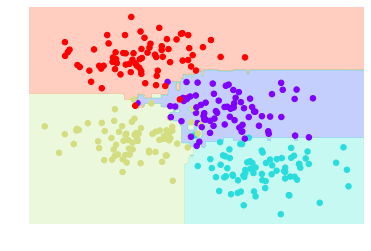In :
tree = DecisionTreeClassifier()
forest = RandomForestClassifier(n_estimators=100)

forest.fit(X, y)
visualize_classifier(forest, X, y)

/Users/Me/anaconda3/lib/python3.7/site-packages/matplotlib/contour.py:1000: UserWarning: The following kwargs were not used by contour: 'clim'
s)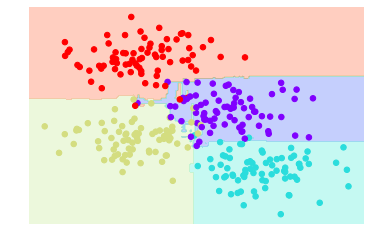#### Categorizing as positive or negative reviews based on vocabulary¶

In :
import nltk

[nltk_data] Downloading package stopwords to /Users/Me/nltk_data...
[nltk_data]   Package stopwords is already up-to-date!

Out:
True
In :
def openFile(path):
#param path: path/to/file.ext (str)
#Returns contents of file (str)
with open(path) as file:
return data
imdb_data = openFile('imdb_labelled.txt')
amzn_data = openFile('amazon_cells_labelled.txt')
yelp_data = openFile('yelp_labelled.txt')

In :
datasets = [imdb_data, amzn_data, yelp_data]

combined_dataset = []
# separate samples from each other
for dataset in datasets:
combined_dataset.extend(dataset.split('\n'))

# separate each label from each sample
dataset = [sample.split('\t') for sample in combined_dataset]

In :
# We now have a list of the form [['review', 'label']],
# A label of '0' indicates a negative sample, while a label of '1' indicates a positive one.
df = pd.DataFrame(data=dataset, columns=['Reviews', 'Labels'])

# Remove any blank reviews
df = df[df["Labels"].notnull()]


Out:
Reviews Labels
0 A very, very, very slow-moving, aimless movie ... 0
1 Not sure who was more lost - the flat characte... 0
2 Attempting artiness with black & white and cle... 0
3 Very little music or anything to speak of. 0
4 The best scene in the movie was when Gerardo i... 1
In :
import string
df['Word Count'] = [len(review.split()) for review in df['Reviews']]

df['Uppercase Char Count'] = [sum(char.isupper() for char in review) \
for review in df['Reviews']]

df['Special Char Count'] = [sum(char in string.punctuation for char in review) \
for review in df['Reviews']]

In :
df.head(10)

Out:
Reviews Labels Word Count Uppercase Char Count Special Char Count
0 A very, very, very slow-moving, aimless movie ... 0 13 1 6
1 Not sure who was more lost - the flat characte... 0 19 1 3
2 Attempting artiness with black & white and cle... 0 31 1 6
3 Very little music or anything to speak of. 0 8 1 1
4 The best scene in the movie was when Gerardo i... 1 21 2 1
5 The rest of the movie lacks art, charm, meanin... 0 20 3 9
6 Wasted two hours. 0 3 1 1
7 Saw the movie today and thought it was a good ... 1 15 1 2
8 A bit predictable. 0 3 1 1
9 Loved the casting of Jimmy Buffet as the scien... 1 10 3 1
In :
from collections import Counter

def getMostCommonWords(reviews, n_most_common, stopwords=None):
# param reviews: column from pandas.DataFrame (e.g. df['Reviews'])
#(pandas.Series)
# param n_most_common: the top n most common words in reviews (int)
# param stopwords: list of stopwords (str) to remove from reviews (list)
# Returns list of n_most_common words organized in tuples as
#('term', frequency) (list)

# flatten review column into a list of words, and set each to lowercase
flattened_reviews = [word for review in reviews for word in \
review.lower().split()]

# remove punctuation from reviews
flattened_reviews = [''.join(char for char in review if \
char not in string.punctuation) for \
review in flattened_reviews]

# remove stopwords, if applicable
if stopwords:
flattened_reviews = [word for word in flattened_reviews if \
word not in stopwords]

# remove any empty strings that were created by this process
flattened_reviews = [review for review in flattened_reviews if review]

return Counter(flattened_reviews).most_common(n_most_common)

In :
negative_samples = df[df['Labels']=="0"]
positive_samples = df[df['Labels']=="1"]

Out:
Reviews Labels Word Count Uppercase Char Count Special Char Count
0 A very, very, very slow-moving, aimless movie ... 0 13 1 6
1 Not sure who was more lost - the flat characte... 0 19 1 3
2 Attempting artiness with black & white and cle... 0 31 1 6
3 Very little music or anything to speak of. 0 8 1 1
5 The rest of the movie lacks art, charm, meanin... 0 20 3 9
In :
from nltk.corpus import stopwords
getMostCommonWords(positive_samples['Reviews'], 10, stopwords.words('english'))
getMostCommonWords(negative_samples['Reviews'], 10, stopwords.words('english'))

Out:
[('bad', 96),
('movie', 94),
('phone', 76),
('dont', 70),
('like', 67),
('one', 67),
('food', 64),
('time', 61),
('film', 57),
('would', 57)]
In :
from sklearn.feature_extraction.text import TfidfVectorizer

In :
vectorizer = TfidfVectorizer(min_df=15)
bow = vectorizer.fit_transform(df['Reviews'])
len(vectorizer.get_feature_names())

Out:
309
In :
from sklearn.model_selection import train_test_split

X_train, X_test, y_train, y_test = train_test_split(bow, labels, test_size=0.33)


### Using Decision Tree¶

In :
classifier = DecisionTreeClassifier()
classifier.fit(X_train,y_train)
classifier.score(X_test,y_test)

Out:
0.704040404040404

### Using Random Forest¶

In :
classifier = RandomForestClassifier()
classifier.fit(X_train,y_train)
classifier.score(X_test,y_test)

Out:
0.7242424242424242

### Searching for best hyperparameters for Random Forest¶

In :
from sklearn.model_selection import RandomizedSearchCV
from scipy import stats

classifier = RandomForestClassifier()

hyperparameters = {
'n_estimators':stats.randint(10,300),
'criterion':['gini','entropy'],
'min_samples_split':stats.randint(2,9),
'bootstrap':[True,False]
}

random_search = RandomizedSearchCV(classifier, hyperparameters, n_iter=65, n_jobs=4)

random_search.fit(bow, labels)

optimized_classifier = random_search.best_estimator_
optimized_classifier.fit(X_train,y_train)

optimized_classifier.score(X_test,y_test)

Out:
0.7515151515151515
In :
negative_sentence = vectorizer.transform(['I hated this product. It is \
not well designed at all, and it broke into pieces as soon as I got it. \
Would not recommend anything from this company.'])

positive_sentence = vectorizer.transform(['The movie was superb - I was \
on the edge of my seat the entire time. The acting was excellent, and the \
scenery - my goodness. Watch this movie now!'])

optimized_classifier.predict_proba(negative_sentence)

Out:
array([[0.68309859, 0.31690141]])
In :
optimized_classifier.predict_proba(positive_sentence)

Out:
array([[0.03169014, 0.96830986]])

### We can write our own sentence and try it out with the random forest model!¶

In [ ]: